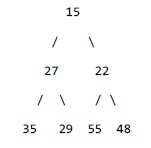# Maximum element in min heap in C++

C++Server Side ProgrammingProgramming

#### C in Depth: The Complete C Programming Guide for Beginners

45 Lectures 4.5 hours

#### Practical C++: Learn C++ Basics Step by Step

Most Popular

50 Lectures 4.5 hours

#### Master C and Embedded C Programming- Learn as you go

66 Lectures 5.5 hours

## Problem statement

Given a minimum heap find maximum element in that.

## Example

If input heap is −Then maximum element is 55

## Algorithm

• In minimum heap parent node will be lesser than its children. Hence we can conclude that a non-leaf node cannot be the maximum.
• Search maximum element in the leaf nodes

## Example

Let us now see an example −

Live Demo

#include <bits/stdc++.h>
using namespace std;
int getMaxElement(int *heap, int n) {
int maxVal = heap[n / 2];
for (int i = n / 2 + 1; i < n; ++i) {
maxVal = max(maxVal, heap[i]);
}
return maxVal;
}
int main() {
int heap[] = {15, 27, 22, 35, 29, 55, 48}; int n = sizeof(heap) / sizeof(heap);
cout << "Maximum element = " << getMaxElement(heap, n) << endl;
return 0;
}

## Output

Maximum element = 55